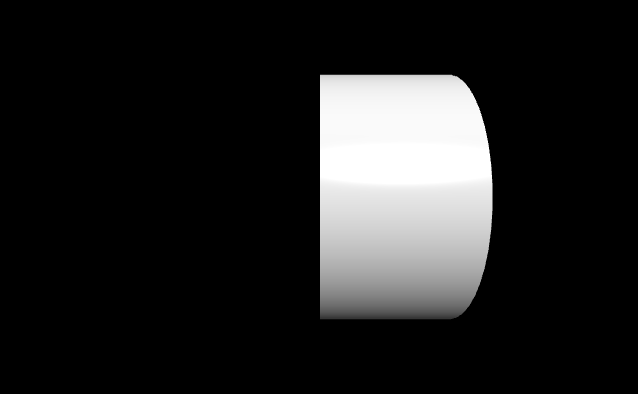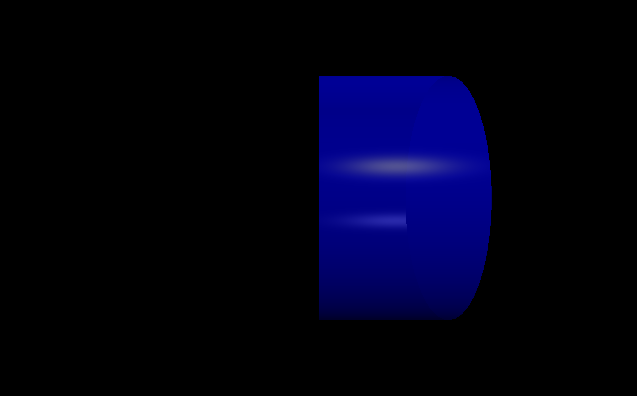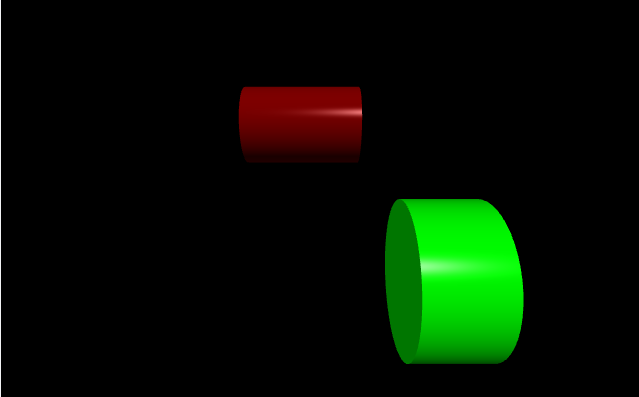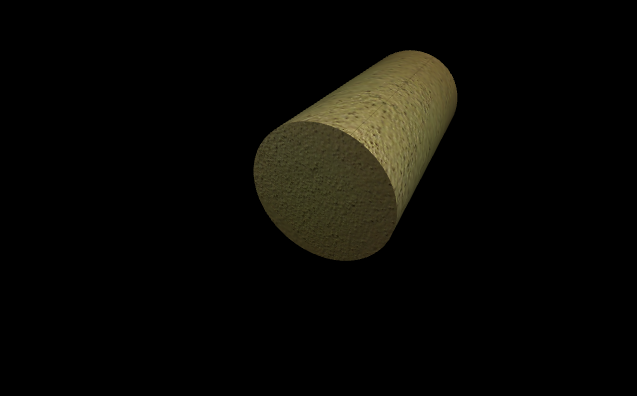# Making a cylinder with VPython

`VPython` makes it easy to create navigable 3D displays and animations, even for those with limited programming experience. Because it is based on Python, it also has much to offer for experienced programmers and researchers. `VPython` allows users to create objects such as spheres and cones in 3D space and displays these objects in a window. This makes it easy to create simple visualizations, allowing programmers to focus more on the computational aspect of their programs. The simplicity of `VPython` has made it a tool for the illustration of simple physics, especially in the educational environment.

Installation :

`pip install vpython`

A cylinder is a geometrical object in three-dimensional space that has straight parallel sides and a circular or oval cross section. We can generate a cone in `VPython` using the `cylinder()` method.

## cylinder()

Syntax : cylinder(parameters)

Parameters :

• pos : It is the position of the center of one end of the cylinder. Assign a vector containing 3 values, example pos = vector(0, 0, 0)
• axis : It is the axis of alignment of the cylinder. Assign a vector containing 3 values, example axis = vector(1, 2, 1)
• up : It is the orientation of the cylinder. Assign a vector containing 3 values, example up = vector(0, 1, 0)
• color : It is the color of the cylinder. Assign a vector containing 3 values, example color = vector(1, 1, 1) will give the color white
• opacity : It is the opacity of the cylinder. Assign a floating value in which 1 is the most opaque and 0 the least opaque, example opacity = 0.5
• shininess : It is the shininess of the cylinder. Assign a floating value in which 1 is the most shiny and 0 the least shiny, example shininess = 0.6
• emissive : It is the emissivity of the cylinder. Assign a boolean value in which True is emissive and False is not emissive, example emissivity = False
• texture : It is the texture of the cylinder. Assign the required texture from the textures class, example texture = textures.stucco
• length : It is the length of the cylinder. Assign a floating value, the default length is 1, example length = 10
• radius : It is the radius of the cylinder. Assign a floating value, the default height is 1, example radius = 5
• size : It is the size of the cylinder. Assign a vector containing 3 values representing the length, height and width respectively, example size = vector(1, 1, 1)

All the parameters are optional.

Example 1 :A cylinder with no parameters, all the parameters will have the default value.

 `# import the module ` `from` `vpython ``import` `*` `cylinder() `

Output :Example 2 :A cylinder using the parameters color, opacity, shininess and emissivity.

 `# import the module ` `from` `vpython ``import` `*` `cone(color ``=` `vector(``0``, ``1``, ``1``),  ` `     ``opacity ``=` `0.5``,  ` `     ``shininess ``=` `1``,  ` `     ``emissive ``=` `False``) `

Output :Example 3 :Displaying 2 cylinders to visualize the attributes pos, length and radius.

 `# import the module ` `from` `vpython ``import` `*` ` `  `# the first cylinder ` `cylinder(pos ``=` `vector(``-``2``, ``2``, ``0``), ` `          ``length ``=` `3``, ` `          ``radius ``=` `1``, ` `          ``color ``=` `vector(``0.5``, ``0``, ``0``)) ` `  `  `# the second cylinder ` `cylinder(pos ``=` `vector(``1``, ``-``1``, ``5``),  ` `          ``color ``=` `vector(``0``, ``1``, ``0``)) `

Output :Example 4 :A cylinder using the parameters texture, axis and up.

 `# import the module ` `from` `vpython ``import` `*` `cylinder(texture ``=` `textures.stucco, ` `         ``axis ``=` `vector(``-``1``, ``4``, ``0``), ` `         ``up ``=` `vector(``1``, ``2``, ``2``)) `

Output :My Personal Notes arrow_drop_upCheck out this Author's contributed articles.

If you like GeeksforGeeks and would like to contribute, you can also write an article using contribute.geeksforgeeks.org or mail your article to contribute@geeksforgeeks.org. See your article appearing on the GeeksforGeeks main page and help other Geeks.

Please Improve this article if you find anything incorrect by clicking on the "Improve Article" button below.

Article Tags :

Be the First to upvote.

Please write to us at contribute@geeksforgeeks.org to report any issue with the above content.